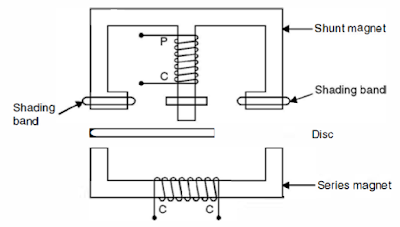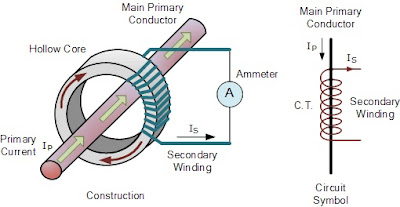## Measuring Instruments

PMMC (Permanent Magnet Moving Coil):Permanent magnet moving coil

Construction:
• It consist light rectangular coil wound on aluminum frame.
• Coil is attached to spindle, which rest on jewelled bearing.
• Soft iron piece is used to reduce the air gap and it makes the magnetic field uniform.
• Two springs are attached to the spindle, they produce control torque and acts as the current leads for the moving coil.
• The pointer moves on a calibrated scale.
• Working:
• When current carrying conductor is placed in the magnetic field, force is exertedover the conductor.
• The magnitude of the force is given by  F=NBIL …………Newtons

When N = the number of turns of coil.
B = Magnetic flux density   wb/m2
I = current flowing through the coil .
L  = length of the conductor cutting the magnetic flux
• The direction of this force is given by Fleming’s left hand rule.
• Statement: keep first three finger of left hand kept perpendicular to each other, first finger indicates direction of magnetic field, middle finger indicated direction of current flowing through the conductor, then thumb indicated the direction of force exerted over conductor.
• Control torque is provided by spring.

MI(moving iron):

Working principle:
• Current to be measured is passed through coil, coil acts as an electromagnet. In attraction type meter this electromagnet attracts the iron piece producing the deflection.
• In attraction type, a small iron piece is attached to the spindle.
• Fixed coil is placed near to the spindle, current is passed through the fixed coil , coil acts as an electromagnet produce magnetic field and iron piece attracted towards the coil.
• The deflecting torque is directly proportional to square of current.
• Hence scale is non-uniform.
• Controlling toque is produced either spring or gravity control.
• Damping torque is produced by air friction damping.
Comparison between PMMC and MI :

 Sr no. PMMC(Permanent Magnet Moving Coil) MI (Moving Iron) 1 It is suitable only for for D.C. Supply It is suitable for AC. as well DC Supply. 2 In PMMC instrument deflecting torque is proportional to current through the coil (TD α I) In MI instrument deflecting torque is proportional to square of current through the coil (TD α I2) 3 Scale is uniform. Scale is non-uniform 3 It is much sensitive than M.I. instruments Less Sensitive as compared to PMMC instruments 4 Torque to weight ratio is high compared to M.I. type Torque to weight ratio is less compared to PMMC type 5 It is costlier than M.I. type It is cheaper than PMMC type. 6 Application: For measuring DC quantities Application: For measuring AC  as well as DC quantities

Induction Type Energy Meter:Induction type energy meter

• Induction type energy meter consist two electromagnets namely shunt magnet and series magnet.
• Shunt magnet connected parallel to the load and series magnet connected in series with the load.
• Aluminum disc  which is kept between the two electromagnets.
• ‘C’ shaped permanent magnet knows as braking magnet, it is fitted near one end of the aluminum disc.
Working:
• When current flows through coil of electromagnet flux is developed.
• Since current is in alternating nature, so flux is changing w.r.t time.
• This flux cuts aluminium disc, hence according to Faraday’s law of electromagnetic induction principle emf get induced in aluminium disc and current start flowing through the disc as result current and the magnetic field produced by the two magnets.
• This produce driving torque and aluminium disc start rotating.
Application of digital multimeter:

1) To measure ac & dc voltages.
2) To measure ac& dc currents.
3) To measure resistances.
4) To check continuity.
5) To test Diode.
6) To test transistor.
7) To measure capacitance.

Clip on ammeter:Clip On Meter

Working of clip on ammeter-
• The principle of operation is shown in above figure, where it can be seen that the Clampon jaws of the instrument act as a transformer Conductor acts as a primary winding.
• The resulting secondary current is then measured by the instrument (taking the turns ratio of the current transformer into account).Current induced in the secondary winding is rectified and applied to a movingcoil meter.
Use:
• Clip-on ammeter is used to measure the AC current without breaking the circuit or
• Without opening the circuit.

Electro-dynamic wattmeter:

Construction:
• It consist two identical fixed coil.
• In the air gap between this fixed coils, moving coil is placed  which is attached to the spindle.
• The fixed coil of this meter is connected in series with load and is knows as current coil of watt meter its terminal are marked as ‘M’ and ‘L’
• The voltage coil is connected parallel with the load,its terminal marked as ‘C’ and ‘V’
Working
• Principle: current carrying moving coil is placed in the magnetic field produced by the two fixed coil, hence force is exerted over the moving coil which is given by
• F=BILsinθ
• So moving coil will become current carrying conductor kept in magnetic field provided by F1 and F2. So moving coil experience a mechanical force and pointer deflected over calibrated scale.